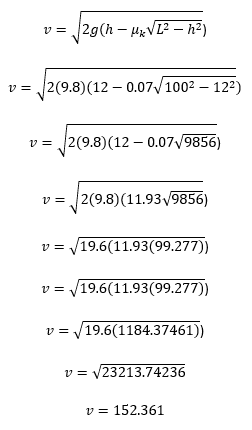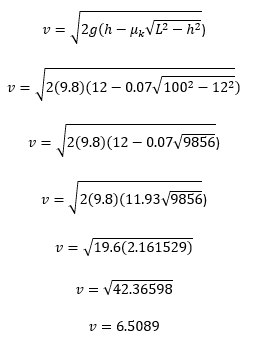# Speed and Kinetic Friction

## Homework Statement

Sam, whose mass is 75 kg, stands at the top of a 12-m-high, 100-m-long snow-covered slope. His skis have a coefficient of kinetic friction on snow of 0.07. If he uses his poles to get started, then glides down, what is his speed at the bottom?

## Homework Equations

v = √(2g(h-μk√(L2-h2)))

## The Attempt at a Solution

It seems as simple as plugging in the values into the equation, but my result isn't anywhere near what it should be.
From my understanding:
g = 9.8
h = 12m
L = 100m
μk = 0.07

v = √(2(9.8)(12-0.07√(1002-122))) = 152.36
152.36 m/s? That seems a bit much to me. The back of my book says that it is 9.9 m/s, but how do I get there?
What did I mess up on?

v = √(2g(h-μk√(L2-h2)))
This is a very specialized formula! (Is this sort of formula supplied for you on exams?)

v = √(2(9.8)(12-0.07√(1002-122))) = 152.36
When I calculate the left side, I don't get 152.36. Make sure you are doing the calculation correctly using your calculator.

•Mastermind01
Yeah we have to memorize it. I have no idea where it came from lol

When I punch it into my calculator I get the same answer. So I tried breaking it down algebraically as follows.
I still ended up with the same weird number.Am I plugging the values into the wrong places?

See if you can spot the error in going from the 3rd to the 4th line. "Order of operations" is important here.

•Rather than multiply, I take the 11.93th root of 9856?When I plug it into my calculator I get the correct answer, but I am concerned that when I do it by hand I am going to mess it up. :/

How would you evaluate ##9 - 2 \cdot 3##

You have a subtraction and a multiplication. Which operation should you do first?

•